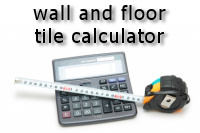# Tile calculator | How many tiles do you need?Welcome to our tile calculator.

The first thing with any tiling project is calculating how many tiles, adhesive and grout you will need to finish the project.

Our calculator will provide you with the estimated amount of tiles required for your tiling project. It will also specify the amount of tile adhesive and grout that you will need to complete the job.

## Calculator instructions

To use our calculator follow these steps:
1. Find the total coverage area.
• For wall tiles measure each wall from left to right (label this X) and from top to bottom (label this Y). Multiply X by Y and note down this figure AR. Repeat for each wall that needs tiling. Finally add all of the AR totals for each wall together and label this figure TCA.
• For floor tiles measure the width (W) and length (L) of the room, remember to include any alcoves. Multiply W by L to give the area required, label this figure TCA.
2. Check the tile dimensions.
• Either measure one of the tiles with your tape measure or check the tile packaging for the dimensions. Label the tile width TW and the tile height TH.
3. Estimate the grout line width.
• This figure is used to estimate the amount of grout required, it will be dependant on the size of tile spacer you intend to use. Label this figure GLW.
4. Entering the values.
• First, use the drop-down selection box to select the measurement system that you have used, choose imperial for measurements given in inches and feet, or metric for milimetres and metres. Enter the TCA value into the "total coverage area" box and enter the TW (tile width) and TH (tile height) figures into their respective tile dimension boxes. Finally, select the GLW (grout line width) from the pull down box provided.
5. Finishing the calculation
• After entering each of the values simply click the "CALCULATE" button to see your estimated figures for tiles, adhesive and grout required. Also, please see our notes at the bottom of this page regarding the estimations given.

## Tile calculator

find the amount of tiles, tile adhesive and grout required for your project
note: all fields are required
Measurement system used
Total coverage area (TCA)
in feet (imperial) or metres (metric)
Tile width (TW)
in inches (imperial) or milimetres (metric)
Tile height (TH)
in inches (imperial) or milimetres (metric)
Grout line width (GLW)
Click below to calculate your estimations

## Calculator estimation notes

Our tile calculator is for informational purposes only. Due to the diversity of individual projects the figures should be taken as a guide only. We do not guarantee that the figures provided will be the exact requirements for the tiling project.

Tile estimation
This figure will be fairly accurate, however, it is calculated by the measurements provided and does not take into account any awkward cuts or tile loss due to damage.